•Why is there no help for me? Whereas all others get their questions answered!

•Gurobi Staff

Hi Rahul,

Why is there no help for me? Whereas all others get their questions answered!

Please note that this is an open voluntary forum. There is no obligation for anyone to post here. Moreover, your post is quite long and detailed with a lot of information to digest making it more complex than an "average" post. Modelling questions can be more difficult to answer as they might require specific knowledge of a problem class. Altogether, it can be expected that an answer to your question might take longer compared to other questions in this forum.

This is where my struggle begins, as from here on, I want to restrict the internal flows between terminals to one is to one flow.

For eg: if terminal 2 needs empty containers, it can either take from terminal 3 or terminal 1 and not both. However, the port can always supply.

When modeling graphs with edges, as you do, one usually defines binary variables for each edge in the graph. A value of $$1$$ means that an edge is active and a value of $$0$$ means that a given edge is not used. You can then define a constraint similar to $$\sum_{i,j} e_{i,j} = 1$$ where you sum over all edges going into a node $$i$$ and force that exactly one of these edges is active. Then, you have to somehow construct a connection between these binaries and your $$S$$ variables. You can achieve this via indicator constraints, where you define "if $$e_{i,j} = 0$$ then $$S_{i,j}=0$$".

I have an additional question. You explicitly set the lower bound of your $$Y$$ variable to $$-\infty$$ but then define constraints which set it $$\geq 0$$. Is this on purpose? Note that this does not invalidate your model, but can be confusing to a reader.

I hope this helps.

Best regards,
Jaromił

•Hi Jaromil,

I agree with you that its not obligatory to reply to one. Thanks for your help.

The reason to not implement binary constraints and then indicator was to avoid long computation time as my actual data is very large. Without using binary constraints and indicator constraints, it is already 350000 variables.
I tried to use this logic:

for j in inland_terminal:    for i in nodes:        for t in time:             if i != j:                                      if               demand[i,j,t]-S[i,t]+Y[j,i,t]-Y[i,j,t] >=0:                        m.addConstr(demand[i,j,t]-S[i,t]+Y[j,i,t]-Y[i,j,t] >=0)                   elif             demand[i,j,t]-S[i,t]+Y[j,i,t]-Y[i,j,t]-Y[port,j,t] >=0:                        m.addConstr(demand[i,j,t]-S[i,t]+Y[j,i,t]-Y[i,j,t]-Y[port,j,t] >=0)


I read that you cannot use Variables in if statements. This generates an error "Constraint has no bool value" over which there is an article as well. Is this logic correct or its wont work in my case?

When referring to binary variable and indicator constraints, modelling them like this would work?

bY = m.addVars(links, lb=0, ub=1, vtype=GRB.BINARY)m.addConstrs(bY.sum(i,j,t)==1 for t in time)for j in inland_terminal:    for i in nodes:        for t in time:            if i != j:                m.addGenConstrIndicator(bY[i,j,t], 'False', S[j,t]==0)

To answer your question about the lower bound n then applying a contradicting variable, that would just be plain human error from my side. The lower bound is not required when defining the variable.

Thanks,

Rahul K

•Gurobi Staff

Hi Rahul,

I read that you cannot use Variables in if statements. This generates an error "Constraint has no bool value" over which there is an article as well. Is this logic correct or its wont work in my case?

It is correct that you cannot use if-statements on optimization variables or expressions. This is because these objects don't have any value before the actual optimization has been executed. From my understanding you would like to enforce the respective constraint only if it's value is >= 0. This can be achieve via conditional statements as discussed in the Knowledge Base article How do I model conditional statements in Gurobi? Note that you will have to introduce additional auxiliary (binary) variables for that.

When referring to binary variable and indicator constraints, modelling them like this would work?

Yes.

You could alternatively add all indicator constraints in one call via

m.addConstrs((bY[i,j,t] == 0) >> (S[j,t] == 0) for j in inland_terminal for i in nodes if i != j for t in time)

Best regards,
Jaromił

•Gurobi Staff

Hi Rahul,

 if demand[i,j,t]-S[i,t]+Y[j,i,t]-Y[i,j,t] >=0:     m.addConstr(demand[i,j,t]-S[i,t]+Y[j,i,t]-Y[i,j,t] >=0) elif demand[i,j,t]-S[i,t]+Y[j,i,t]-Y[i,j,t]-Y[port,j,t] >=0:     m.addConstr(demand[i,j,t]-S[i,t]+Y[j,i,t]-Y[i,j,t]-Y[port,j,t] >=0)

Adding them as conditional statements into the model does not make sense, because they would read "if x >= 0 then enforce x >= 0" which does have any effect. It would just over-complicate the model formulation.

Best regards,
Jaromił

•Hej,

I have tried everything I could understand from the documentation and other posts in the community however, the objective function for the model is higher that what it should be. I have solved the manually and the solution is different that the one provided by Gurobi. The flow is not getting optimized the way it should. There are possibilities to further reduce the cost but the model does not recognize them. I am guessing it is a problem with the constraints but I am not able to figure out where.

This is the code:

supply = {('port', 'terminal1', datetime.date(2022, 1, 2)): 0,
('port', 'terminal1', datetime.date(2022, 1, 3)): 14,
('port', 'terminal1', datetime.date(2022, 1, 4)): 11,
('port', 'terminal1', datetime.date(2022, 1, 5)): 91,
('port', 'terminal1', datetime.date(2022, 1, 6)): 26,
('port', 'terminal1', datetime.date(2022, 1, 7)): 89,
('port', 'terminal1', datetime.date(2022, 1, 8)): 46,
('port', 'terminal1', datetime.date(2022, 1, 9)): 10,
('port', 'terminal2', datetime.date(2022, 1, 2)): 0,
('port', 'terminal2', datetime.date(2022, 1, 3)): 0,
('port', 'terminal2', datetime.date(2022, 1, 4)): 2,
('port', 'terminal2', datetime.date(2022, 1, 5)): 7,
('port', 'terminal2', datetime.date(2022, 1, 6)): 4,
('port', 'terminal2', datetime.date(2022, 1, 7)): 9,
('port', 'terminal2', datetime.date(2022, 1, 8)): 0,
('port', 'terminal2', datetime.date(2022, 1, 9)): 13,
('port', 'terminal3', datetime.date(2022, 1, 2)): 0,
('port', 'terminal3', datetime.date(2022, 1, 3)): 0,
('port', 'terminal3', datetime.date(2022, 1, 4)): 3,
('port', 'terminal3', datetime.date(2022, 1, 5)): 2,
('port', 'terminal3', datetime.date(2022, 1, 6)): 1,
('port', 'terminal3', datetime.date(2022, 1, 7)): 0,
('port', 'terminal3', datetime.date(2022, 1, 8)): 7,
('port', 'terminal3', datetime.date(2022, 1, 9)): 0}demand = {('port', 'terminal1', datetime.date(2022, 1, 2)): 0,
('port', 'terminal1', datetime.date(2022, 1, 3)): 6,
('port', 'terminal1', datetime.date(2022, 1, 4)): 0,
('port', 'terminal1', datetime.date(2022, 1, 5)): 19,
('port', 'terminal1', datetime.date(2022, 1, 6)): 16,
('port', 'terminal1', datetime.date(2022, 1, 7)): 4,
('port', 'terminal1', datetime.date(2022, 1, 8)): 0,
('port', 'terminal1', datetime.date(2022, 1, 9)): 0,
('port', 'terminal2', datetime.date(2022, 1, 2)): 0,
('port', 'terminal2', datetime.date(2022, 1, 3)): 29,
('port', 'terminal2', datetime.date(2022, 1, 4)): 4,
('port', 'terminal2', datetime.date(2022, 1, 5)): 46,
('port', 'terminal2', datetime.date(2022, 1, 6)): 22,
('port', 'terminal2', datetime.date(2022, 1, 7)): 0,
('port', 'terminal2', datetime.date(2022, 1, 8)): 18,
('port', 'terminal2', datetime.date(2022, 1, 9)): 0,
('port', 'terminal3', datetime.date(2022, 1, 2)): 0,
('port', 'terminal3', datetime.date(2022, 1, 3)): 32,
('port', 'terminal3', datetime.date(2022, 1, 4)): 40,
('port', 'terminal3', datetime.date(2022, 1, 5)): 43,
('port', 'terminal3', datetime.date(2022, 1, 6)): 0,
('port', 'terminal3', datetime.date(2022, 1, 7)): 45,
('port', 'terminal3', datetime.date(2022, 1, 8)): 0,
('port', 'terminal3', datetime.date(2022, 1, 9)): 0}cost = {('port', 'terminal1'): 120,
('terminal1', 'port'): 120,
('port', 'terminal2'): 489,
('terminal2', 'port'): 489,
('port', 'terminal3'): 257,
('terminal3', 'port'): 257,
('terminal1', 'terminal2'): 371,
('terminal1', 'terminal3'): 214. ('terminal2', 'terminal1'): 371, ('terminal2', 'terminal3'): 277, ('terminal3', 'terminal1'): 214, ('terminal3', 'terminal2'): 277}#Setsnodes = ['port', 'terminal1','terminal2','terminal3']inland_terminals = ['terminal1', 'terminal2' 'terminal3']time = ([date(2022,1,2), date(2022,1,3), date(2022,1,4), date(2022,1,5), date(2022,1,6), date(2022,1,7), date(2022,1,8), date(2022,1,9)])links = list(permutations(nodes,2))blinks = list(permutations(inland_terminal,2))#Variablesflow = m.addVars(links, time, vtype = GRB.INTEGER)flow_bin = m.addVars(blinks,time, vtype = GRB.BINARY)#Constraintsm.addConstrs(flow[i,j,t] >= 0 for i,j,t in flow)# to have active flow in only one arcm.addConstrs((flow_bin.sum(i,j) <= 1 for i in nodes for j in nodes if i!=j))# indicator constraintsfor i in inland_terminal:    for j in inland_terminal:        if i != j:            m.addConstrs((flow_bin[i,j,t] == 0) >> (flow[i,j,t] == 0) for t in time)                        for i in inland_terminal:    for j in inland_terminal:        if i != j:            m.addConstrs((flow_bin[i,j,t] == 1) >> (sum((flow[i, j, t] - sum(flow[j, i ,t]for i in inland_terminal if i!=j)) for i in inland_terminal if i !=j)>= 0) for t in time)# the flow constraint to ensure that the demand is metm.addConstrs(supply['GHAB', j, t] + flow['port', j, t] + sum((flow[i, j, t]- sum(flow[j, i ,t] for i in inland_terminal if i !=j)) for i in inland_terminal if i !=j) >= demand['port', j, t] for i in inland_terminal for j in inland_terminal if i !=j for t in time)#objective functionm.setObjective(sum((flow[i,j,t]*cost[i,j]) for i,j,t in flow), GRB.MINIMIZE)m.update()m.optimize()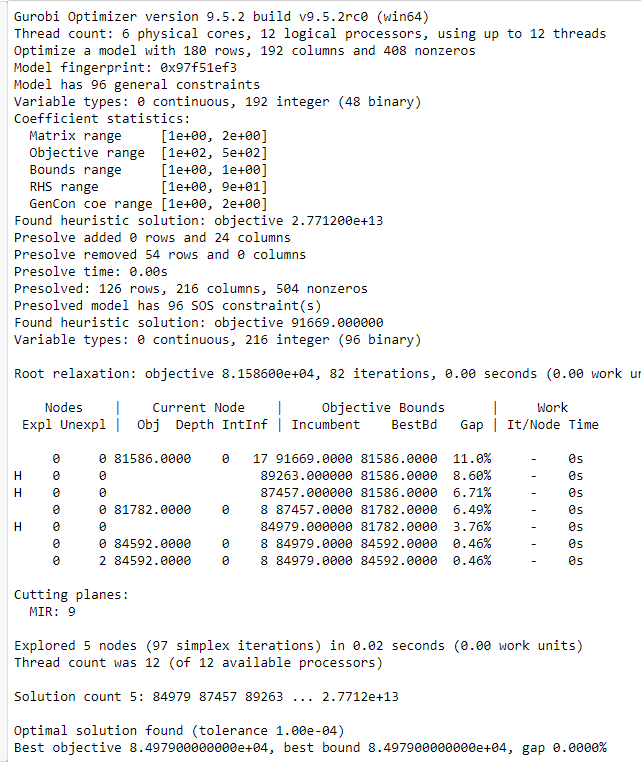The cost here is 84979. When calculated by hand, the cost is around 75200.

When I check the variable values after optimization, the port is sending containers, even though other terminals are closer to the destination and have spare containers. If somehow this is resolved, then the cost will reduce.

Thank you for help in advance. Any and all inputs will be highly appreciated.

•Gurobi Staff

You could try fixing all variables to the values of your optimal solution. You can do this by setting the variable's lower and upper bound to the same value. For example

x.lb = 1x.ub = 1

would fix variable $$x$$ to value $$1$$.

Please note that default lower bounds for variables are $$0$$. Thus, if your integer variables can attain negative values, then you have to explicitly define it via

flow = m.addVars(links, time, lb=-GRB.INFINITY, vtype = GRB.INTEGER)

If the model becomes infeasible, you can then proceed as described in the Knowledge Base article How do I determine why my model is infeasible?

If the solution point is indeed feasible then you might want to post the solution point computed by hand as well.

•This is how I calculated it and checked for the lowest possible cost. The port has infinite supply. However, the terminal can only accept from one other terminal and the port. A terminal can send to many but as mentioned above only receive from one. The cost is around 79000 and not 75000.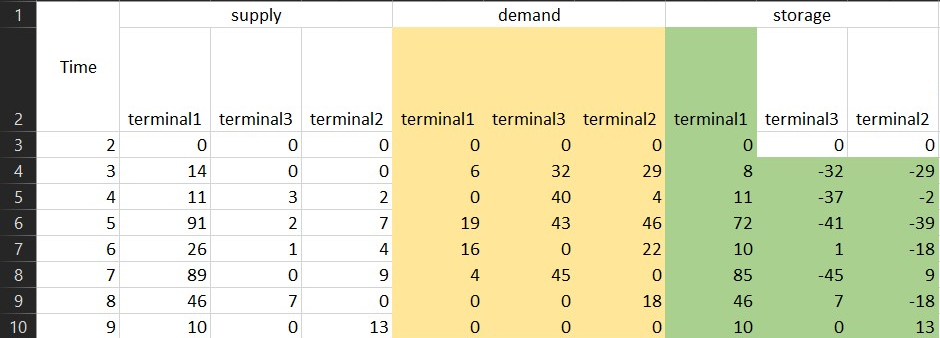The negative sign simply means that there is a deficit of so many containers.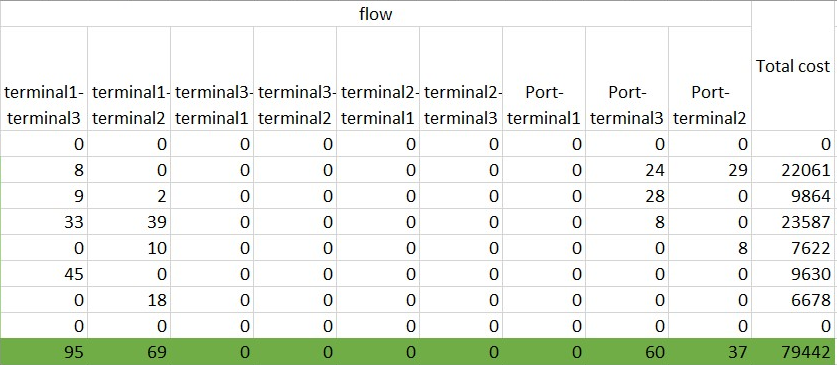With the above suggestion of setting the variable flow to -infinity, while keeping the condition as >= in the flow constraint it results the model being unbounded. I am not sure about the first suggestion you gave for

fixing variables to the values of your optimal solution.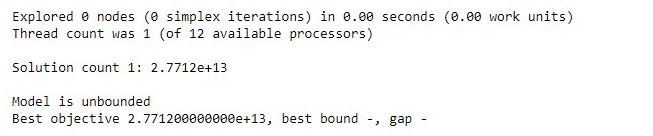If I change the condition in flow constraint to == then the model is infeasible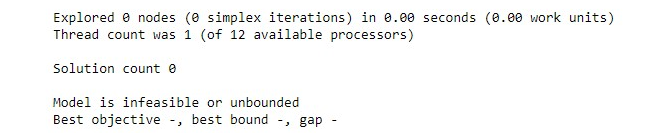Further I check with m.computeIIS(), which says cannot be computed for a feasible model.

Now, I tried by changing the condition here from <= to == and setting the ub=79442, the IIS says that there is 1 constraint.

# to have active flow in only one arc
m.addConstrs((flow_bin.sum(i,j) == 1 for i in nodes for j in nodes if i!=j))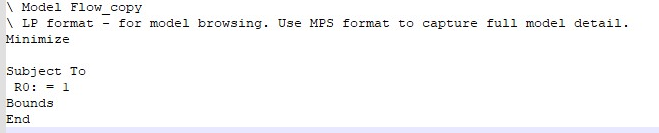•Gurobi Staff

I am not sure I understand what exactly you did you get the outputs you show.

By fixing variable values, I mean to manually add equality constraints for every variable fixing it to what you think should be the optimal solution value. In your case this would mean adding

m.addConstr(flow[i,j,t] == XXX) # do this for every i j in nodes t in timem.addConstr(flow_bin[i,j,t] == XXX) # do this for every i j in nodes t in time

This way, your model cannot be unbounded, because all variables are fixed to certain value. It can however be infeasible.

You should write your model to a file using the write method.

m.setObjective(sum((flow[i,j,t]*cost[i,j]) for i,j,t in flow), GRB.MINIMIZE)
m.update()
m.optimize()m.write("myModel.lp")

You can then open the file $$\texttt{myModel.lp}$$ in any standard text editor and check whether the model actually looks like what it should.

Please note that the code snippet you posted has multiple errors and is not executable, e.g, you define $$\texttt{inland_terminals}$$ but use $$\texttt{inland_terminal}$$ later on, Model $$\texttt{m}$$ is not defined, there is a $$\texttt{.}$$ instead of $$\texttt{,}$$ in the $$\texttt{cost}$$ dictionary.

•Hej Jaromil,

I just went through the code again and made sure its executable. I will add the bounds individually and check and get back to you. However, will I have to do it individually if the problem has many more variables? Because in the current problem there are 216 integer variables of which 96 are binary. It will take a lot of time to add so many lines.

# Librariesimport mathimport numpy as npimport pandas as pdfrom itertools import permutationsimport gurobipy as gpfrom gurobipy import GRB, Model, quicksum, multidictfrom datetime import *import openpyxlsupply = {('port', 'terminal1', date(2022, 1, 2)): 0, ('port', 'terminal1', date(2022, 1, 3)): 14, ('port', 'terminal1', date(2022, 1, 4)): 11, ('port', 'terminal1', date(2022, 1, 5)): 91, ('port', 'terminal1', date(2022, 1, 6)): 26, ('port', 'terminal1', date(2022, 1, 7)): 89, ('port', 'terminal1', date(2022, 1, 8)): 46, ('port', 'terminal1', date(2022, 1, 9)): 10, ('port', 'terminal2', date(2022, 1, 2)): 0, ('port', 'terminal2', date(2022, 1, 3)): 0, ('port', 'terminal2', date(2022, 1, 4)): 2, ('port', 'terminal2', date(2022, 1, 5)): 7, ('port', 'terminal2', date(2022, 1, 6)): 4, ('port', 'terminal2', date(2022, 1, 7)): 9, ('port', 'terminal2', date(2022, 1, 8)): 0, ('port', 'terminal2', date(2022, 1, 9)): 13, ('port', 'terminal3', date(2022, 1, 2)): 0, ('port', 'terminal3', date(2022, 1, 3)): 0, ('port', 'terminal3', date(2022, 1, 4)): 3, ('port', 'terminal3', date(2022, 1, 5)): 2, ('port', 'terminal3', date(2022, 1, 6)): 1, ('port', 'terminal3', date(2022, 1, 7)): 0, ('port', 'terminal3', date(2022, 1, 8)): 7, ('port', 'terminal3', date(2022, 1, 9)): 0}demand = {('port', 'terminal1', date(2022, 1, 2)): 0, ('port', 'terminal1', date(2022, 1, 3)): 6, ('port', 'terminal1', date(2022, 1, 4)): 0, ('port', 'terminal1', date(2022, 1, 5)): 19, ('port', 'terminal1', date(2022, 1, 6)): 16, ('port', 'terminal1', date(2022, 1, 7)): 4, ('port', 'terminal1', date(2022, 1, 8)): 0, ('port', 'terminal1', date(2022, 1, 9)): 0, ('port', 'terminal2', date(2022, 1, 2)): 0, ('port', 'terminal2', date(2022, 1, 3)): 29, ('port', 'terminal2', date(2022, 1, 4)): 4, ('port', 'terminal2', date(2022, 1, 5)): 46, ('port', 'terminal2', date(2022, 1, 6)): 22, ('port', 'terminal2', date(2022, 1, 7)): 0, ('port', 'terminal2', date(2022, 1, 8)): 18, ('port', 'terminal2', date(2022, 1, 9)): 0, ('port', 'terminal3', date(2022, 1, 2)): 0, ('port', 'terminal3', date(2022, 1, 3)): 32, ('port', 'terminal3', date(2022, 1, 4)): 40, ('port', 'terminal3', date(2022, 1, 5)): 43, ('port', 'terminal3', date(2022, 1, 6)): 0, ('port', 'terminal3', date(2022, 1, 7)): 45, ('port', 'terminal3', date(2022, 1, 8)): 0, ('port', 'terminal3', date(2022, 1, 9)): 0}cost = {('port', 'terminal1'): 120, ('terminal1', 'port'): 120, ('port', 'terminal2'): 489, ('terminal2', 'port'): 489, ('port', 'terminal3'): 257, ('terminal3', 'port'): 257, ('terminal1', 'terminal2'): 371, ('terminal1', 'terminal3'): 214, ('terminal2', 'terminal1'): 371, ('terminal2', 'terminal3'): 277, ('terminal3', 'terminal1'): 214, ('terminal3', 'terminal2'): 277}#Setsnodes = ['port', 'terminal1','terminal2','terminal3']inland_terminal = ['terminal1', 'terminal2', 'terminal3']time = ([date(2022,1,2), date(2022,1,3), date(2022,1,4), date(2022,1,5), date(2022,1,6), date(2022,1,7), date(2022,1,8), date(2022,1,9)])links = list(permutations(nodes,2))blinks = list(permutations(inland_terminal,2))m = gp.Model('Flow')#Variablesflow = m.addVars(links, time, vtype = GRB.INTEGER)flow_bin = m.addVars(blinks,time, vtype = GRB.BINARY)#Constraintsm.addConstrs(flow[i,j,t] >= 0 for i,j,t in flow)# to have active flow in only one arcm.addConstrs((flow_bin.sum(i,j) <= 1 for i in nodes for j in nodes if i!=j))# indicator constraintsfor i in inland_terminal:    for j in inland_terminal:        if i != j:            m.addConstrs((flow_bin[i,j,t] == 0) >> (flow[i,j,t] == 0) for t in time)                        for i in inland_terminal:    for j in inland_terminal:        if i != j:            m.addConstrs((flow_bin[i,j,t] == 1) >> (sum((flow[i, j, t] - sum(flow[j, i ,t]for i in inland_terminal if i!=j)) for i in inland_terminal if i !=j)>= 0) for t in time)# the flow constraint to ensure that the demand is metm.addConstrs(supply['port', j, t] + flow['port', j, t] + sum((flow[i, j, t]- sum(flow[j, i ,t] for i in inland_terminal if i !=j)) for i in inland_terminal if i !=j) >= demand['port', j, t] for i in inland_terminal for j in inland_terminal if i !=j for t in time)#objective functionm.setObjective(sum((flow[i,j,t]*cost[i,j]) for i,j,t in flow), GRB.MINIMIZE)m.update()m.optimize()m.write("myModel.lp")
•Gurobi Staff

However, will I have to do it individually if the problem has many more variables?

You somehow have to provide this information to Gurobi. If you have the optimal solution point as some data structure or a file, then you could try to automate at least some part of the process by reading the data structure or file and set the respective variable in a loop.

•I have the optimal solution in this case because its a small part of a larger dataset. In the actual data set there are 300000 variables and I do not have the optimal solution for the larger dataset because its impossible to solve by hand.

m.addConstr(flow_bin[i,j,t] == XXX) # do this for every i j in nodes t in time

In this part, the I want to constrain the flow from inland terminals. Such that only one of them should be able to provide containers. If I were to do it for every i j in nodes then port will be included which will not model it the way I want. So should I do it for every i j in inland terminal only in order to keep the flow from port active always but only keep one arc active from inland terminals?

•Gurobi Staff

In this part, the I want to constrain the flow from inland terminals. Such that only one of them should be able to provide containers. If I were to do it for every i j in nodes then port will be included which will not model it the way I want. So should I do it for every i j in inland terminal only in order to keep the flow from port active always but only keep one arc active from inland terminals?

Sorry, but I am not sure I fully understand the issue. Are you stating that the solution which you think should be optimal for the particular model you posted here is actually a subset of a way bigger solution to a way bigger model? Wouldn't it then be possible that some relationships from the bigger model are missing in the smaller model which ultimately does not allow for the optimal solution value you want?

You could also try fixing a part of your variables of which you think are necessary to construct the optimal solution you have in mind. This should hopefully be enough to debug this issue.

•Hej Jaromil,

I am saying that the code above is a small part of a larger dataset which has data with dates ranging over half a year with many more inland terminals. Where I have to optimize the entire network instead of just the 3 inland terminals as the code above.

Some good news finally, I made progress with your suggestions on constraining each variable and that results in the same solution and matches with what I calculated by hand. I had to make a change in the equation though. Previously, I used this as you can see in the above code, which made the model infeasible now after adding the individual constraints.

# the flow constraint to ensure that the demand is metm.addConstrs(supply['port', j, t] + flow['port', j, t]+ sum((flow[i, j, t]- sum(flow[j, i ,t]for i in inland_terminal if i !=j) for i in inland_terminal if i !=j)) >= demand['port', j, t] for i in inland_terminal for j in inland_terminal if i !=j for t in time)

Thus, I changed it to this instead and it gives me the exact solution.

m.addConstrs(supply['port', j, t] + flow['port', j, t]+ sum((flow[i, j, t]- flow[j, i ,t] ) for i in inland_terminal if i !=j) >= demand['port', j, t] for i in inland_terminal for j in inland_terminal if i !=j for t in time)

However, I have a few questions regarding the modelling it self.

Question 1:

When modelling the binary constraint for flow, should I model it as this

for t in time:    m.addConstr((flow_bin.sum(i,j,t) <= 1))

or as this

for t in time:    m.addConstr((flow_bin.sum(i,j,t) == 1))

The difference being the condition used. In the 2nd option, the model becomes infeasible and I suppose it so happens that for some t in time, there are no flows anywhere in the entire network and thus the infeasibility.

Question 2:

When modelling the flow constraint: the equation for satisfying demand. Should I model it using ">=" or "==". I have modeled it as:

# the flow constraint to ensure that the demand is metm.addConstrs(supply['port', j, t] + flow['port', j, t] + sum((flow[i, j, t]- flow[j, i ,t] ) for i in inland_terminal if i !=j) >= demand['port', j, t] for i in inland_terminal for j in inland_terminal if i !=j for t in time)

Or should I model it as:

# the flow constraint to ensure that the demand is metm.addConstrs(supply['port', j, t] + flow['port', j, t] + sum((flow[i, j, t]- flow[j, i ,t] ) for i in inland_terminal if i !=j) == demand['port', j, t] for i in inland_terminal for j in inland_terminal if i !=j for t in time)

Again when using "==" the model becomes infeasible and I am not able to make sense of it.

•supply = {('port', 'terminal1', date(2022, 1, 2)): 0, ('port', 'terminal1', date(2022, 1, 3)): 14, ('port', 'terminal1', date(2022, 1, 4)): 11, ('port', 'terminal1', date(2022, 1, 5)): 91, ('port', 'terminal1', date(2022, 1, 6)): 26, ('port', 'terminal1', date(2022, 1, 7)): 89, ('port', 'terminal1', date(2022, 1, 8)): 46, ('port', 'terminal1', date(2022, 1, 9)): 10, ('port', 'terminal2', date(2022, 1, 2)): 0, ('port', 'terminal2', date(2022, 1, 3)): 0, ('port', 'terminal2', date(2022, 1, 4)): 2, ('port', 'terminal2', date(2022, 1, 5)): 7, ('port', 'terminal2', date(2022, 1, 6)): 4, ('port', 'terminal2', date(2022, 1, 7)): 9, ('port', 'terminal2', date(2022, 1, 8)): 0, ('port', 'terminal2', date(2022, 1, 9)): 13, ('port', 'terminal3', date(2022, 1, 2)): 0, ('port', 'terminal3', date(2022, 1, 3)): 0, ('port', 'terminal3', date(2022, 1, 4)): 3, ('port', 'terminal3', date(2022, 1, 5)): 2, ('port', 'terminal3', date(2022, 1, 6)): 1, ('port', 'terminal3', date(2022, 1, 7)): 0, ('port', 'terminal3', date(2022, 1, 8)): 7, ('port', 'terminal3', date(2022, 1, 9)): 0}demand = {('port', 'terminal1', date(2022, 1, 2)): 0, ('port', 'terminal1', date(2022, 1, 3)): 6, ('port', 'terminal1', date(2022, 1, 4)): 0, ('port', 'terminal1', date(2022, 1, 5)): 19, ('port', 'terminal1', date(2022, 1, 6)): 16, ('port', 'terminal1', date(2022, 1, 7)): 4, ('port', 'terminal1', date(2022, 1, 8)): 0, ('port', 'terminal1', date(2022, 1, 9)): 0, ('port', 'terminal2', date(2022, 1, 2)): 0, ('port', 'terminal2', date(2022, 1, 3)): 29, ('port', 'terminal2', date(2022, 1, 4)): 4, ('port', 'terminal2', date(2022, 1, 5)): 46, ('port', 'terminal2', date(2022, 1, 6)): 22, ('port', 'terminal2', date(2022, 1, 7)): 0, ('port', 'terminal2', date(2022, 1, 8)): 18, ('port', 'terminal2', date(2022, 1, 9)): 0, ('port', 'terminal3', date(2022, 1, 2)): 0, ('port', 'terminal3', date(2022, 1, 3)): 32, ('port', 'terminal3', date(2022, 1, 4)): 40, ('port', 'terminal3', date(2022, 1, 5)): 43, ('port', 'terminal3', date(2022, 1, 6)): 0, ('port', 'terminal3', date(2022, 1, 7)): 45, ('port', 'terminal3', date(2022, 1, 8)): 0, ('port', 'terminal3', date(2022, 1, 9)): 0}cost = {('port', 'terminal1'): 120, ('terminal1', 'port'): 120, ('port', 'terminal2'): 489, ('terminal2', 'port'): 489, ('port', 'terminal3'): 257, ('terminal3', 'port'): 257, ('terminal1', 'terminal2'): 371, ('terminal1', 'terminal3'): 214, ('terminal2', 'terminal1'): 371, ('terminal2', 'terminal3'): 277, ('terminal3', 'terminal1'): 214, ('terminal3', 'terminal2'): 277}#Setsnodes = ['port', 'terminal1','terminal2','terminal3']inland_terminal = ['terminal1', 'terminal2', 'terminal3']time = ([date(2022,1,2), date(2022,1,3), date(2022,1,4), date(2022,1,5), date(2022,1,6), date(2022,1,7), date(2022,1,8), date(2022,1,9)])links = list(permutations(nodes,2))blinks = list(permutations(inland_terminal,2))#list for defining general constraintspt1 = list([0,0,0,0,0,0,0,0])pt2 = list([0,29,0,0,8,0,0,0])pt3 = list([0,24,28,8,0,0,0,0])t1t2 = list([0,0,2,39,10,0,18,0])t1t3 = list([0,8,9,33,0,45,0,0])t1p = list([0,0,0,0,0,0,0,0])t2t1 = list([0,0,0,0,0,0,0,0])t2t3 = list([0,0,0,0,0,0,0,0])t2p = list([0,0,0,0,0,0,0,0])t3t1 = list([0,0,0,0,0,0,0,0])t3t2 = list([0,0,0,0,0,0,0,0])t3p = list([0,0,0,0,0,0,0,0])bin_pt1 = list([0,0,0,0,0,0,0,0])bin_pt2 = list([0,1,0,0,1,0,0,0])bin_pt3 = list([0,1,1,1,0,0,0,0])bin_t1t2 = list([0,0,1,1,1,0,1,0])bin_t1t3 = list([0,1,1,1,0,1,0,0])bin_t2t1 = list([0,0,0,0,0,0,0,0])bin_t2t3 = list([0,0,0,0,0,0,0,0])bin_t3t1 = list([0,0,0,0,0,0,0,0])bin_t3t2 = list([0,0,0,0,0,0,0,0]) m = gp.Model('Flow')#Variablesflow = m.addVars(links, time, lb=-GRB.INFINITY, vtype = GRB.INTEGER)flow_bin = m.addVars(links,time, vtype = GRB.BINARY)#Constraints# to have active flow in only one arcfor t in time:    m.addConstr((flow_bin.sum(i,j,t) <= 1))# indicator constraintsfor i in inland_terminal:    for j in inland_terminal:        if i != j:            m.addConstrs((flow_bin[i,j,t] == 0) >> (flow[i,j,t] == 0) for t in time)                        for i in inland_terminal:    for j in inland_terminal:        if i != j:            m.addConstrs((flow_bin[i,j,t] == 1) >> (sum((flow[i, j, t] - flow[j, i ,t]) for i in inland_terminal if i !=j)>= 0) for t in time)#general constraintsfor t in range(len(time)):    m.addConstr(flow['port','terminal1', time[t]] == pt1[t])    m.addConstr(flow['port','terminal2', time[t]] == pt2[t])    m.addConstr(flow['port','terminal3', time[t]] == pt3[t])    m.addConstr(flow['terminal1','port', time[t]] == t1p[t])    m.addConstr(flow['terminal1','terminal2', time[t]] == t1t2[t])    m.addConstr(flow['terminal1','terminal3', time[t]] == t1t3[t])    m.addConstr(flow['terminal2','port', time[t]] == t2p[t])    m.addConstr(flow['terminal2','terminal1', time[t]] == t2t1[t])    m.addConstr(flow['terminal2','terminal3', time[t]] == t2t3[t])    m.addConstr(flow['terminal3','port', time[t]] == t3p[t])    m.addConstr(flow['terminal3','terminal1', time[t]] == t3t1[t])    m.addConstr(flow['terminal3','terminal2', time[t]] == t3t2[t])#general binary constraintsfor t in range(len(time)):    m.addConstr(flow_bin['port','terminal1',time[t]] == bin_pt1[t])    m.addConstr(flow_bin['port','terminal2',time[t]] == bin_pt2[t])    m.addConstr(flow_bin['port','terminal3',time[t]] == bin_pt3[t])    m.addConstr(flow_bin['terminal1','terminal2',time[t]] == bin_t1t2[t])    m.addConstr(flow_bin['terminal1','terminal3',time[t]] == bin_t1t3[t])    m.addConstr(flow_bin['terminal2','terminal1',time[t]] == bin_t2t1[t])    m.addConstr(flow_bin['terminal2','terminal3',time[t]] == bin_t2t3[t])    m.addConstr(flow_bin['terminal3','terminal1',time[t]] == bin_t3t1[t])    m.addConstr(flow_bin['terminal3','terminal2',time[t]] == bin_t3t2[t])# the flow constraint to ensure that the demand is metm.addConstrs(supply['port', j, t] + flow['port', j, t]+ sum((flow[i, j, t]- flow[j, i ,t] ) for i in inland_terminal if i !=j) >= demand['port', j, t] for i in inland_terminal for j in inland_terminal if i !=j for t in time)#objective functionm.setObjective(sum((flow[i,j,t]*cost[i,j]) for i,j,t in flow), GRB.MINIMIZE)m.update()m.optimize()#m.computeIIS()m.write("myModel.lp")#m.write("m.ilp")
•Gurobi Staff

Question 1:

When modelling the binary constraint for flow, should I model it as this

for t in time:
m.addConstr((flow_bin.sum(i,j,t) <= 1))

or as this

for t in time:
m.addConstr((flow_bin.sum(i,j,t) == 1))

The difference being the condition used. In the 2nd option, the model becomes infeasible and I suppose it so happens that for some t in time, there are no flows anywhere in the entire network and thus the infeasibility.

It depends on what you want to enforce. I would guess that going with $$\leq 1$$ should be correct as there can be a time window where no flow is present for a certain time $$t$$. In this particular $$\texttt{sum}$$, what are you actually adding up? It looks like there is a $$\texttt{for}$$-loop missing.

Question 2:

When modelling the flow constraint: the equation for satisfying demand. Should I model it using ">=" or "==". I have modeled it as:

# the flow constraint to ensure that the demand is met
m.addConstrs(supply['port', j, t] + flow['port', j, t] + sum((flow[i, j, t]- flow[j, i ,t] ) for i in inland_terminal if i !=j) >= demand['port', j, t] for i in inland_terminal for j in inland_terminal if i !=j for t in time)

Or should I model it as:

# the flow constraint to ensure that the demand is met
m.addConstrs(supply['port', j, t] + flow['port', j, t] + sum((flow[i, j, t]- flow[j, i ,t] ) for i in inland_terminal if i !=j) == demand['port', j, t] for i in inland_terminal for j in inland_terminal if i !=j for t in time)

Again when using "==" the model becomes infeasible and I am not able to make sense of it.

Here going with equality constraint can definitely be too restrictive. This is because all your variables are discrete and it is possible that there is no combination to fulfill the constraint exactly with equality. A$$\leq$$ inequality also fulfills the demand and allows for overshooting which avoids enforcing impossible discrete combinations.

•Hej Jaromil,

I have solved this problem. Also, you point of correctly that there is a for loop missing which I fixed. The model is running now and works perfectly.

This is how I fixed the for loop problem

for j in inland_terminal:     for t in time:        m.addConstr( sum(flow_bin[i,j,t]  for i in inland_terminal if i!=j) <= 1)

what are you actually adding up?

I was trying to get constraints like these:

R1: flow_bin[terminal1,terminal2,2022-01-03]
+ flow_bin[terminal1,terminal3,2022-01-03] <= 1
R2: flow_bin[terminal2,terminal3,2022-01-03]
+ flow_bin[terminal1,terminal3,2022-01-03] <= 1
R3: flow_bin[terminal2,terminal3,2022-01-03]
+ flow_bin[terminal1,terminal3,2022-01-03] <= 1

I think the answer to my questions are in line with what I was thinking. However, I have posted another question in a separate post related to sum of constraints.

I sincerely thank you for all the help and inputs. Have a great day.

•Hej Jaromil,

I think I was too quick to dismiss the problem thinking that I got it cleared. It just does not seem to work. I have been trying to strictly restrict flow in arcs and it always does something crazy.

I want to restrict internal flows in the network which leads to transhipment of containers. Transhipment meaning that the containers are sent to a node where it is not required but then further sent to another node. This reduces overall cost but it does not meet the requirements I have. I just need flows if its possible otherwise not.

In the image below,

The demand at terminal5 is for 6 containers. There is no supply on that date to terminal5. What I need is that that it receives containers from one of the other terminals if available otherwise from the port.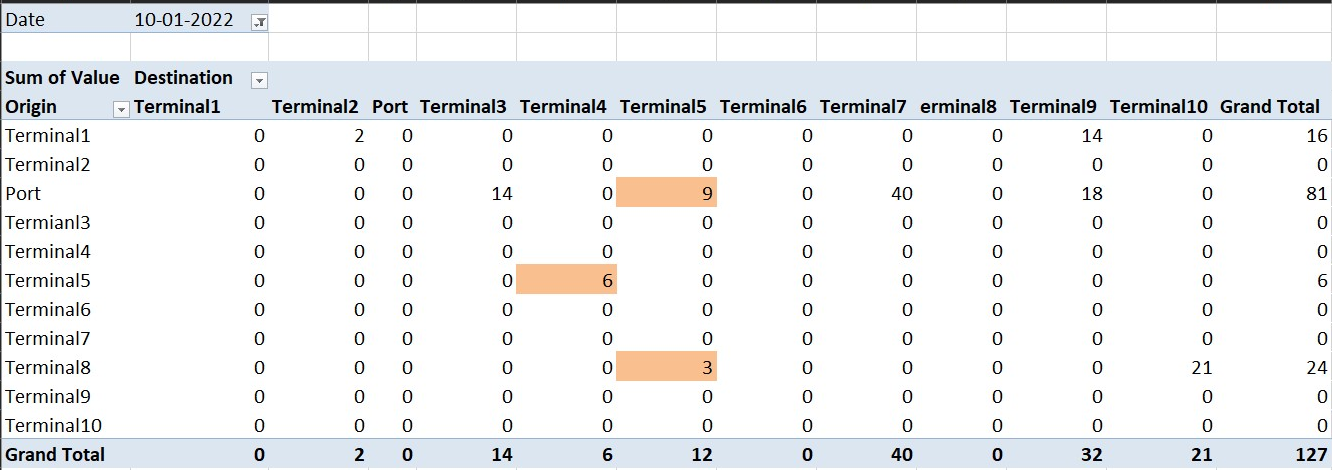As you can see here, even though terminal5 needs containers but it is also sending 6 to terminal4. This is because of the transhipment. In this case the transhipment is because

Terminal5 receives a total of 12 containers where 9 are from port and 3 are from terminal8(which should not happen), whereas it should receive only 6(either from one terminal or port). Then it takes the extra 6 and send it to the terminal4.

I used this code to produce the Indicator Constraints:

for j in inland_terminal:    for t in time:                m.addConstr(sum(flow_bin[i,j,t] for i in inland_terminal if i!=j) <= 1)#indicator constraints 1for i in inland_terminal:    for j in inland_terminal:        if i != j:            m.addConstrs((flow_bin.sum(i,j,t) == 0) >> (flow.sum(i,j,t) == 0) for t in time)#indicator constraint 2 v.1for i in inland_terminal:    for j in inland_terminal:        for t in time:            if i != j:                m.addConstr(((flow_bin.sum(i,j,t)) == 1) >> (flow[j,i,t]>=0))

I have tried another version of indicator constraint 2.

#indicator constraint 2 v.2for i in inland_terminal:    for j in inland_terminal:        for t in time:            if i != j:                m.addConstr(((flow_bin.sum(i,j,t)) == 1) >> (sum(flow[i,j,t] - flow[j, i ,t])>=0))

Using the 2nd version leads to this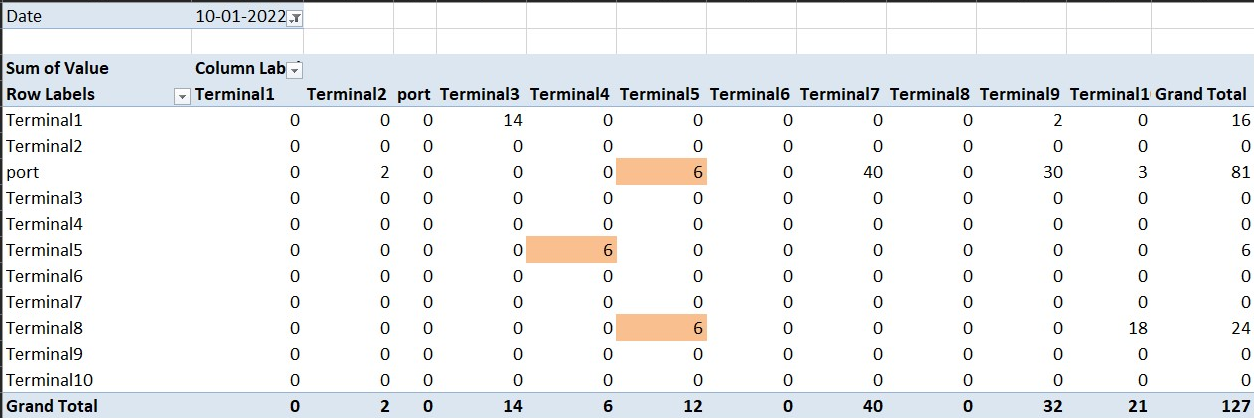•Gurobi Staff

Terminal5 receives a total of 12 containers where 9 are from port and 3 are from terminal8(which should not happen), whereas it should receive only 6(either from one terminal or port). Then it takes the extra 6 and send it to the terminal4.

I have no expertise about shipping applications, but Is it really an issue if terminal 5 receives 12 containers of which it ships 6 further? Couldn't it be better this way, e.g., if the distance for shipping from port and terminal8 to terminal 5 and then 6 to terminal4 is indeed shorter than shipping from port to terminal4 directly?

In your indicator constraints, you are trying to take the sum

m.addConstrs((flow_bin.sum(i,j,t) == 0) >> (flow.sum(i,j,t) == 0) for t in time)

but you are actually using only one particular $$\texttt{flow_bin}$$ and $$\texttt{flow}$$ value, because $$\texttt{i,j,t}$$ are fixed during the loop iteration. If you want to model a constraint of the form

\begin{align*} \sum_{i=0}^N f^{bin}_i &= 0 \rightarrow \sum_{i=0}^L f_i = 0\quad(*)\\ f^{bin}_i,f_i &\in \{0,1\} \end{align*}

then you have to proceed differently. You have to introduce an auxiliary binary variable $$b$$ and big-M constraints

\begin{align*} \sum_{i=0}^N f^{bin}_i &\geq 0.5 + M\cdot b - M\\ \sum_{i=0}^N f^{bin}_i &\leq M\cdot b \end{align*}

This way, if $$\sum_{i=0}^N f^{bin}_i=0$$ then $$b=0$$ and $$b=1$$ otherwise. The value of the big-M can be chosen as $$N$$ which equals the total number of $$f^{bin}_i$$ variables in the summation in term $$(*)$$.

You can then introduce an indicator constraint

\begin{align*} b = 0 \rightarrow \sum_{i=0}^M f_i = 0 \end{align*}

You would have to introduce the auxiliary binary variables and big-M constraints for every such condition $$(*)$$ that you have to introduce. You can find more details about conditional statements in Gurobi in the Knowledge Base article How do I model conditional statements in Gurobi?

Best regards,
Jaromił

•Hej Jaromil,

Couldn't it be better this way, e.g., if the distance for shipping from port and terminal8 to terminal 5 and then 6 to terminal4 is indeed shorter than shipping from port to terminal4 directly?

What you point out is absolutely right and would be the best way to minimize cost. That would be true in the case of a transport problem with flows possible in a many-to-many format. However, in my case, the destination can only receive from one but can send out to many. I am checking if triangulation is possible or not between the port and a pair of inland terminals.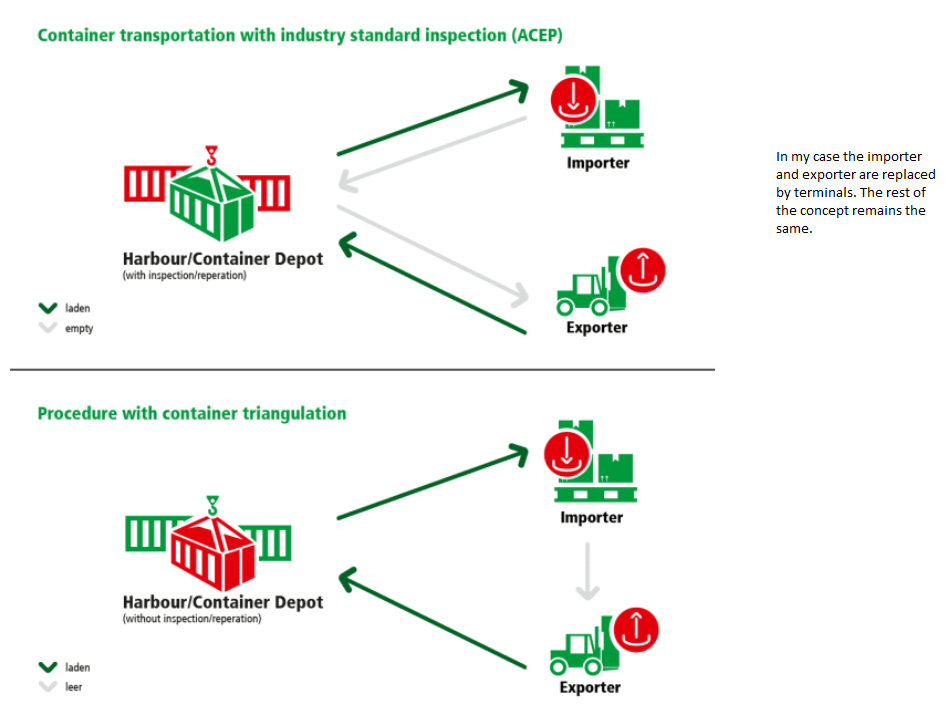I was hoping that it does not come down to the big M constraint, but looks like there is no other way to proceed. I will work on it and post here when I come across an issue. Thank you for the help Jaromil.

•Hej Jaromil,

If you want to model a constraint of the form

\begin{align*}
\sum_{i=0}^N f^{bin}_i &= 0 \rightarrow \sum_{i=0}^L f_i = 0\quad(*)\\
f^{bin}_i,f_i &\in \{0,1\}
\end{align*}

You have written it as the above equation, but in my case it should be something like this, will that change the procedure or will I have to move ahead with big M and auxiliary binary variables?

\begin{align*}
\sum_{i=0}^N f^{bin}_i &= 0 \rightarrow \sum_{i=0}^N f_i = 0\quad(*)\\
f^{bin}_i &\in \{0,1\}\\ f_i & \in \{\mathbb{N}\}\end{align*}

•Gurobi Staff

The only difference is than $$L$$ is $$N$$ and $$f_i \in \mathbb{N}$$ in your formulation, is this correct? Then no, nothing changes compared to my last response.

•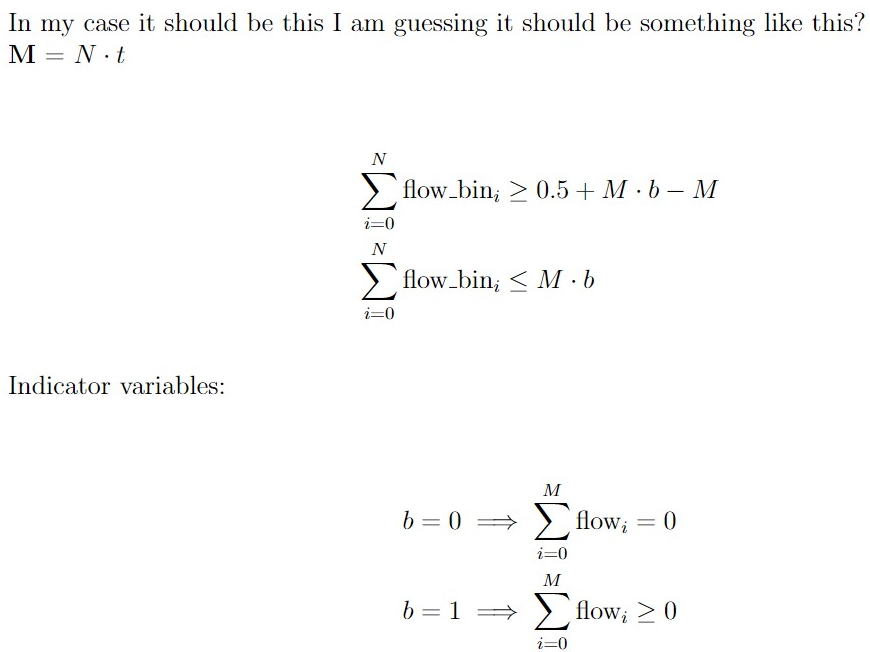for j in inland_terminal:    for t in time:                m.addConstr(sum(flow_bin[i,j,t] for i in inland_terminal if i!=j) <= 1)#constantsM = 21for j in inland_terminal:    for t in time:        m.addConstr(sum(flow_bin[i,j,t] for i in inland_terminal if i!=j) >= 0.5 + (M*b) - b)        for j in inland_terminal:    for t in time:        m.addConstr(sum(flow_bin[i,j,t] for i in inland_terminal if i!=j) <= (M * b))for i in inland_terminal:    for j in inland_terminal:        if i != j:            m.addConstrs((b == 0) >> (flow.sum(i,j,t) == 0) for t in time)for i in inland_terminal:    for j in inland_terminal:        for t in time:            if i != j:                m.addConstr((b == 1) >> (sum(flow[i,j,t] - flow[j, i ,t] for i in inland_terminal if i!=j) >=0))

I am using M = N .t because my total number of variables when there are 3 elements in and 7 elements in  is 21 variables. This I cross check from the .lp file and it matches what I need. So, in that case will M = 21 work for me? Also, I loop them over j and t because I need it like that. This leads to an infeasible model though.

The m.computeIIS() gives this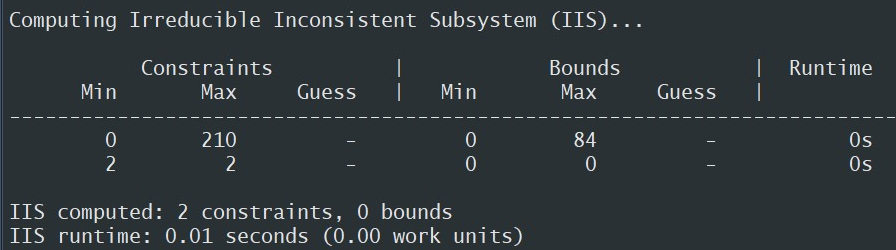Another question is, why the 0.5? Is that because my flow variable is an integer and not a continuous variable?

•Gurobi Staff
m.addConstr(sum(flow_bin[i,j,t] for i in inland_terminal if i!=j) >= 0.5 + (M*b) - b)

The right hand side should read $$\texttt{0.5 + (M*b) - M}$$.

Another question is, why the 0.5? Is that because my flow variable is an integer and not a continuous variable?

Yes, for a continuous variable, you would need to define a small $$\epsilon > 0$$, but since your variables are binary, $$0.5$$ does the job perfectly.

In the model.lp file there are two sets of constraints which look like this for some reason!

m.addConstr(sum(flow_bin[i,j,t] for i in inland_terminal if i!=j) >= 0.5 + (M*b) - b)

and

m.addConstr(sum(flow_bin[i,j,t] for i in inland_terminal if i!=j) <= (M * b))

You should always give your constraints unique names to better recognize them when writing files.

This I cross check from the .lp file and it matches what I need. So, in that case will M = 21 work for me?

Yes, $$M=21$$ should work in this case.

•Hello again,

So all of that got sorted out but the same problem again as it was before we introduced big M constraints. Its still doing transhipment instead of taking it from port. Could it be because of the way flow_bin variable is modelled? Because I tried 3 different types:

#Type 1 code and result below:for j in inland_terminal:    for t in time:                m.addConstr(sum(flow_bin[i,j,t] for i in inland_terminal if i!=j) <= 1)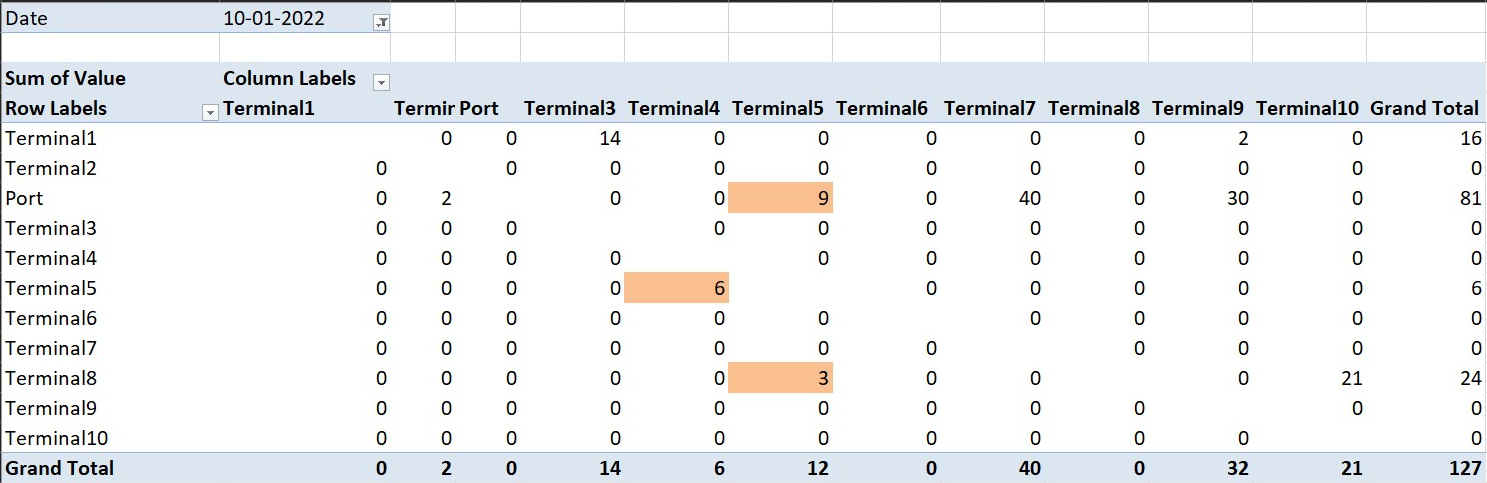#Type 2 code and result below:for j in inland_terminal:    for t in inland_terminal:        m.addConstr((flow_bin.sum('*',j,t)<= 1))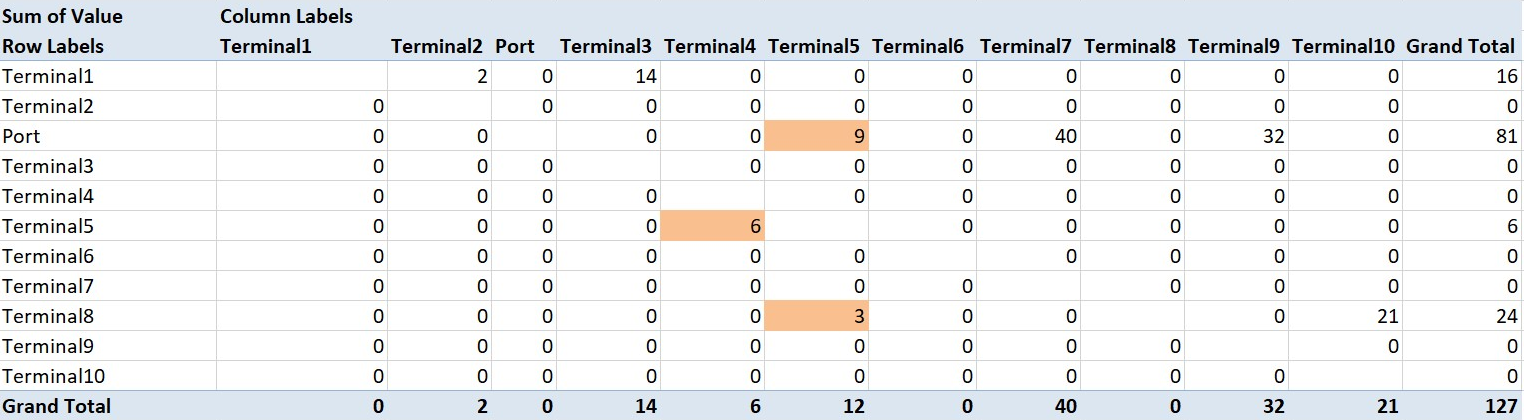Type1 and Type 2 produce the same result because instead of using sum(), I use " * " which results in the same. However, type3 produces what I need but results in flows only from the port.

#Type 3 code and result belowfor j in inland_terminal:    for t in inland_terminal:        m.addConstr((flow_bin.sum('*',j,'*')<= 1))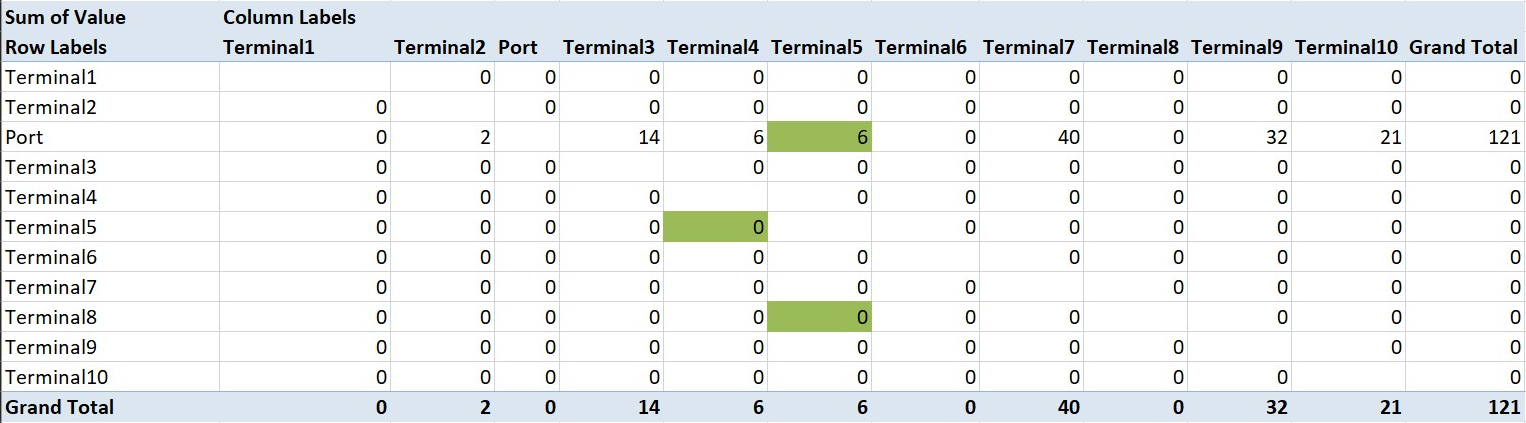As you can see there are no extra flows leading to transhipment in type3. Also in the right lower corner you see that the total number of containers have reduced as compared to type1 and type2. So as you had pointed out about the cost being lower while using shipment, in my case we consider that the cost of having an extra container is higher than the extra cost incurred due to distance travelled.

Now, after introducing the big M constraint, I use type 3 with M=1991 as I have 11 elements in N and 181 in t , it doesnt take many iterations but provides an answer where there is no flow internally but only from the port. This also gives an optimal value around 7.5 million.

I know that the type3 way of modelling it is wrong because it changes the math itself.

With type1 way of modelling along with big M constraints, I get an optimal value of 5.9 million after 12235 simplex iterations but it results in transhipment.

Any insights on this aspect? Thank you.

•Gurobi Staff

Any insights on this aspect?

In the first two versions, you fix the time index for each constraint. In the third version you add a constraint summing over all times. I would recommend to give each constraint a meaningful name and then write each version to an LP file via the write method. Then, you can analyze each version of these constraints and try to find out what is happening.

•Hej Jaromil,

Now I can confirm that it worked finally without any transhipment. I had to add another constraint to make it work. Thank you for everything and wish you a great weekend.

Regards,

Rahul K